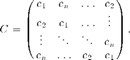# Building a Circulant Operator

guide_circulant

In our first example we will use Spot to create an implicit circulant matrix and that can be used as a fast operator. Circulant matrices are fully specified by their first column, and each remaining column is a cyclic permutation of the first:These matrices have the property that they can be diagonalized by the discrete Fourier transform (DFT), so that

```C = F'diag(d)F,
```

where d = Fc. An important implication is that C can be treated as a fast operator.

## Instantiating a simple Spot operator

In our toy example, we create a circulant matrix whose first column is simply the sequence 1,...,5:

```n = 5;
c = (1:n)';  % make sure c is a column vector
```

Our very first Spot command will create the required DFT operator. We omit the semicolon so that Matlab will display details of the resulting operator:

```F = opDFT(n)
```
```F =
Spot operator: DFT(5,5)
rows:      5    complex: yes
cols:      5    type:    DFT
```

It's straightforward to apply the resulting DFT operator to a vector. The following command applies the Fourier transform to the vector c, which yields the eigenvalues of C:

```d = sqrt(n)*F*c
```
```d =
15.0000 + 0.0000i
-2.5000 + 3.4410i
-2.5000 + 0.8123i
-2.5000 - 0.8123i
-2.5000 - 3.4410i
```

Notice that we scaled this product by sqrt(n). This is necessary because Spot's DFT is scaled so that it's orthogonal, and therefore, rather than the first column of F being a vector of ones, it's scaled by 1/sqrt(n). We can see this as follows:

```sqrt(n) * double( F(:,1) )
```
```ans =
1
1
1
1
1
```

This last command hints at an important feature of Spot operators that allows them to be indexed like explicit Matlab matrices.

## Building more complex operators

Finally, we build the circulant operator with the command

```C = real( F'*opDiag(d)*F )
```
```C =
Spot operator: Real(DFT(5,5)' * Diag(5,5) * DFT(5,5))
rows:      5    complex: no
cols:      5    type:    real
```

We could have left out the real modifier, and used the simpler command

```C = F'*opDiag(d)*F
```

However, we need to safeguard against numerical errors that might allow complex values to seep in. Hence, we finish off our construction with the real operator.

Just as the DFT operator F could be applied to vectors, the same is true of the compound operator C. Here we apply it to the first unit vector:

```e = eye(5,1);
C*e
```
```ans =
1.0000
2.0000
3.0000
4.0000
5.0000
```

Let's count the number of times that C and F were applied to vectors:

```C.nprods
```
```ans =
1     0
```
```F.nprods
```
```ans =
3     1
```

The suffix nprods gives the number of times that the operator acted on a vector: the first element of X.nprods tracks the count of products with the operator; the second element tracks the count of products with its adjoint. In the first case, we see that C was only used once (in the command C*e above). In the second case, we see that F acted on a vector a total of 4 times---3 with F itself, and once with its adjoint. Where did these 4 products come from? The first is clear, and happened in the command d = sqrt(n)*F*c. Another multiplication with F occurred in the command double( F(:,1) ), which extracted the first column from F. Then two more multiplications, one with F and another with F' occurred when we applied C*e.

Let's verify that C is indeed circulant. We can do this two ways. The first uses the overloaded method double which converts a Spot operator into an explicit matrix:

```double(C)
```
```ans =
1.0000    5.0000    4.0000    3.0000    2.0000
2.0000    1.0000    5.0000    4.0000    3.0000
3.0000    2.0000    1.0000    5.0000    4.0000
4.0000    3.0000    2.0000    1.0000    5.0000
5.0000    4.0000    3.0000    2.0000    1.0000
```

The second approach shows that it's possible to apply an operator simultaneously to a collection of vectors:

```C*eye(n)
```
```ans =
1.0000    5.0000    4.0000    3.0000    2.0000
2.0000    1.0000    5.0000    4.0000    3.0000
3.0000    2.0000    1.0000    5.0000    4.0000
4.0000    3.0000    2.0000    1.0000    5.0000
5.0000    4.0000    3.0000    2.0000    1.0000
```